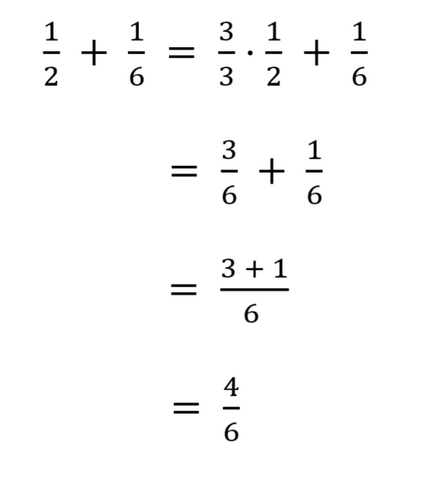# Solving Word Fraction Problems

hesi a2 math

Fractions can get pretty hairy if you are unsure on how to break down a word problem. The HESI A2 math section is FILLED with fractions.

It's kind of like that quote from Forest Gump, except it's more like: adding fractions, subtracting fractions, dividing fractions, multiplying fraction, percentages and fractions, rations and fractions, etc.

Craig has 1/2 cup of juice. Joe has 1/6 cup more of juice than Craig. How much juice does Joe have?

Solution: Joe has 1/2 + 1/6 cup of juice. We need to calculate the sum. Notice that the denominators are different.

Step 1: Find the least common denominator:

• 2, 4, 6, 8, 10, 12
• 6, 12, 18, 24, 30, 36

So LCM (2,6) = 6

Step 2-3: Convert 1/2 and 1/6 into equivalent simple fractions with the common denominator equal to 6 and calculate their sum.Answer: Joe has 4/6 cup of juice (when reduced) then he has 2/3 cup of juice.

To get a free HESI math practice test with fully explained answers and solutions, enter your email below.

• whyeah on

i failed the hesi 3 x, need help with math ection please, thanks